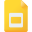# logarithmic functions tutorialsExponential and Logarithmic Equations; Further Applications
Logarithmic Functions, High School, United States, MathematicsProperties of Logarithms
Logarithmic Functions, High School, United States, MathematicsCommon and Natural Logarithms
Logarithmic Functions, High School, United States, MathematicsDefine Logarithmic Functions
Logarithmic Functions, High School, United States, MathematicsDerivatives of Logarithmic Functions
Derivative, High School, United States, Mathematics, Logarithmic FunctionsLogistic Growth Model of an Exponential Function
Logarithmic Functions, High School, United States, Integral Calculus, Mathematics